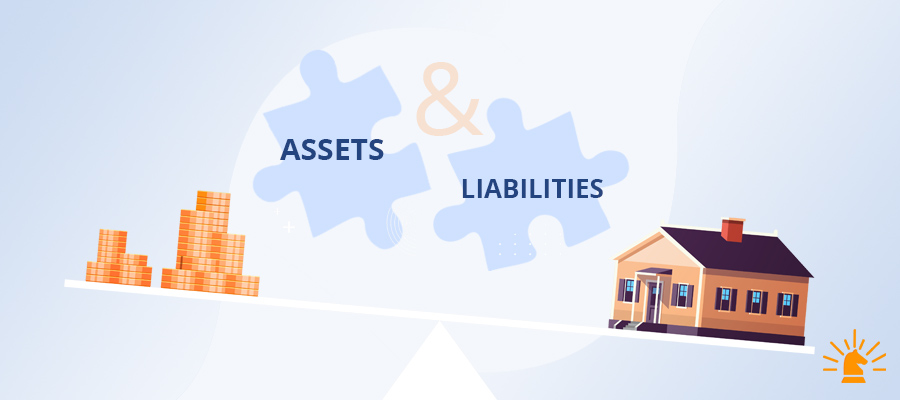## Asset Liability Management (ALM)###### Updated on Oct 12, 2020 | By Author Sakshi Shekhawat

While building its ALM policy, a bank or financial institution can use capping the percentage of exposure in each category against the total balance sheet size. An illustrative example of this balance sheet mix limit mechanism is shown below.

In January 2013, the Basel Committee has issued the full text of the revised Liquidity Coverage Ratio (LCR) as one of the key components of the Basel III capital framework. This new ratio was introduced so as to ensure that banks will have sufficient capital adequacy.

While the balance sheet is considered as the main focus while undertaking the exercise of the ALM process, it is not all.  Banks often enter into transactions that are not recorded in the balance sheet or accounts. These are known as the off-balance-sheet (OBS) transactions. Such transactions are done for extending non-fund based facilities to their clients and generating profits through leveraged positions. It is also done to manage the risk on the balance sheet. A bank’s off-balance exposures comprise of guarantees, letters of credit, derivatives contracts, etc.

After the deregulation of interest rate and with the introduction of new instruments and products, the traditional Asset Liability Management process has undergone a several changes. Mismatch of Asset and Liability in various ways may affect the bank’s viability. With the global turmoil of 2008, slow down in the growth of our economy, and rising off-balance sheet exposure of the banks has become very important to explore the interrelation between the asset sides and the liability side of the balance sheet.

#### Techniques of ALM

1. GAP Analysis Model
2. Duration Model
3. SImulation
4. VaR

#### GAP Analysis Model

This model measures the direction and extent of asset-liability mismatch through either funding or maturity gap. It is computed for assets and liabilities of differing maturities and is calculated for a set time period. This model looks at the gap that exists between the interest revenue earned on the bank's assets and the interest paid on its liabilities over a particular period of time. This is also known as the net interest income exposure of the bank.

A positive gap indicates that assets are greater than liabilities, whereas, a negative gap indicates that liabilities are greater than the assets. The latter is worrisome.

∆NII = (RSAs - RSLs) x ∆r
∆NII = GAP x ∆r

Here, the NII refers to the net interest income, R refers to the interest rates that impact the assets and liabilities in the relevant maturity bucket and GAP is simply the differences between the rate-sensitive assets (RSA) and the rate-sensitive liabilities (RSL). Thus, using this analysis, one can easily identify the impact of the change on the net interest income of the bank, when the interest rates change.

The gap is according to the various time buckets. The positive gap indicates that it has more RSA than RSL whereas the negative gap indicates that it has more RSL. The gap report indicates how the bank or institution will get impacted with change in interest rates. For those having a Positive Gap (RSA > RSL) benefit from a rise in interest rates and vice versa.

#### Duration Model

Duration is an important tool that measures the interest rate sensitivity of assets and liabilities as it takes into account the time of arrival of cash flows and the maturity of assets and liabilities. Duration basically refers to the average life of the asset or liability.

DP p = D (dR /1+R)

#### Simulation

Simulation models introduce a dynamic element while doing the analysis of the interest rate risk. Gap analysis and duration analysis as stand-alone tools for asset-liability management suffer from their inability to move beyond the static analysis of current interest rate risk exposures. Basically simulation models utilize computer power to reconstruct the banking portfolio and running what-if scenarios.

Value At Risk

This refers to the maximum expected loss that a bank can suffer over a target horizon, given a certain confidence interval. It helps to calculate the market risk of a portfolio for which no historical data exists. It is often used for measuring the market risk of a portfolio of assets and/or liabilities.